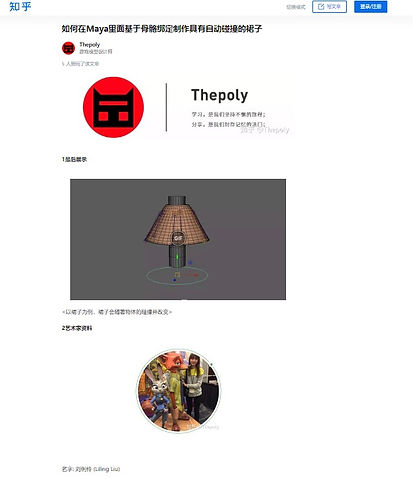top of page# "How to Create A Joint-Based Skirt Rig With Auto Collision In The Maya"- Tutorial

The concept is during of letting object one collides with another object two, they start to collide. First, make a circle which simply represents the structure of the skirt into inside the skirt. Second, when the object one is moving, need to know how the distance and angle are between with the circle point. Using this angle to collide object two. Also, it needs to know how the object two and circle point’s distance to get the collision.

4 main sections.

1. Calculate the distance between an object and the circle

2. Calculate the distance between skirt and the circle

3. Determine the distance between object and skirt to collide

4. Calculate the angle of the object to collide